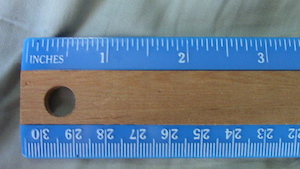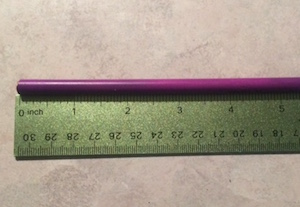How to Use a Ruler in Inches

Instructor: Julie Zundel

Julie has taught high school Zoology, Biology, Physical Science and Chem Tech. She has a Bachelor of Science in Biology and a Master of Education.

What do all of the little lines on ruler represent? How do you measure using inches? Don't worry, this lesson will answer all of your inch-measuring questions.

Metric vs. Standard Measurement

Let's say you want to measure something. Do you find yourself using miles, inches and feet or kilometers, centimeters and meters? If it's the first, you are using the standard measurement system, sometimes referred to as English measurement, which is the measurement system primarily used in the United States. Take a moment to look at the table below comparing some measurements in the standard system to the metric system. The metric system, by the way, is the measurement system used in most of the world and by scientists in the United States.

Measurement Standard Metric
Weight Pounds Kilogram
Volume Quart Liter
Length Inches Centimeter

Using a Ruler

The focus of this lesson is measuring using the standard system and inches, which is a measurement of length. Specifically, there are 12 inches in one foot. Before we begin, you will need to fetch a ruler. If you take a look at the ruler, you will see that there are often two sides. One side uses the metric system, whereas the other side uses the standard system.Before you start measuring anything, make sure you are using the correct side. Remember, for this lesson you are using inches. Some rulers, like the ruler in the above image, have a label that says 'inches,' 'inch' or 'in.' If you do not see any of these labels, look for the side that has numbers one through 12 (since there are typically 12 inches on a ruler).

Next, align the object you want to measure with the left side of the ruler. Rulers read from left to right.If you take a look at the ruler you'll see a lot of little lines and then bigger lines with numbers. Each number represents an inch. Each little line is 1/16th of an inch. So the first is 1/16th, the second is 2/16ths (which reduces to 1/8th), and so and on so forth. Take a look at the table to see what each line represents.

To unlock this lesson you must be a Study.com Member.

Register to view this lesson

Are you a student or a teacher?